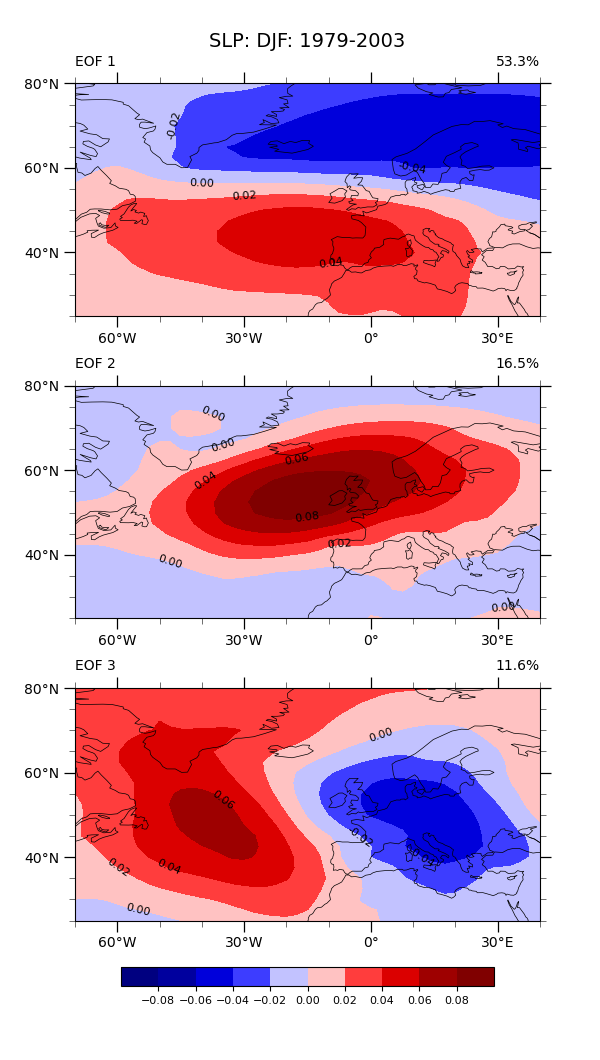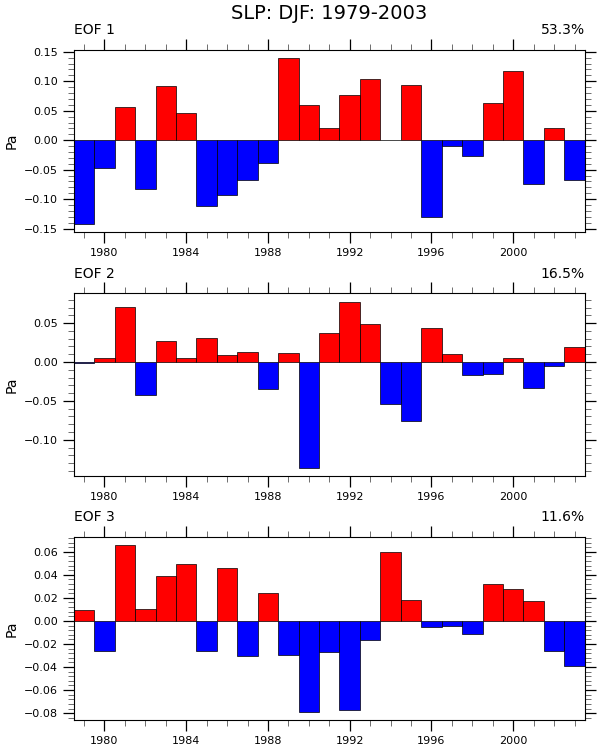# NCL_eof_1_1.py#

Calculate EOFs of the Sea Level Pressure over the North Atlantic.

This script illustrates the following concepts:
• Calculating EOFs

• Drawing a time series plot

• Using coordinate subscripting to read a specified geographical region

• Rearranging longitude data to span -180 to 180

• Calculating symmetric contour intervals

• Drawing filled bars above and below a given reference line

• Drawing subtitles at the top of a plot

• Reordering an array

See following URLs to see the reproduced NCL plot & script:
Note (1):

So-called original NCL plot “eof_1_2_lg.png” given in the above URL is likely not identical to what the given NCL original script generates. When the given NCL script is run, it generates a plot with identical data to that is plotted by this Python script.

Import packages:

```import xarray as xr
import numpy as np
import matplotlib.pyplot as plt
import cartopy.crs as ccrs
import cmaps

import geocat.datafiles as gdf
import geocat.viz as gv
from geocat.comp import eofunc_eofs, eofunc_pcs, month_to_season
```

User defined parameters and a convenience function:

```# In order to specify region of the globe, time span, etc.
latS = 25.
latN = 80.
lonL = -70.
lonR = 40.

yearStart = 1979
yearEnd = 2003

neof = 3  # number of EOFs
```

```# Open a netCDF data file using xarray default engine and load the data into xarrays
ds = xr.open_dataset(gdf.get('netcdf_files/slp.mon.mean.nc'))
```
```/home/docs/checkouts/readthedocs.org/user_builds/geocat-examples/conda/latest/lib/python3.10/site-packages/xarray/coding/times.py:153: SerializationWarning: Ambiguous reference date string: 1-1-1 00:00:0.0. The first value is assumed to be the year hence will be padded with zeros to remove the ambiguity (the padded reference date string is: 0001-1-1 00:00:0.0). To remove this message, remove the ambiguity by padding your reference date strings with zeros.
warnings.warn(warning_msg, SerializationWarning)
```

Flip and sort longitude coordinates:

```# To facilitate data subsetting

ds["lon"] = ((ds["lon"] + 180) % 360) - 180

# Sort longitudes, so that subset operations end up being simpler.
ds = ds.sortby("lon")
```

Place latitudes in increasing order:

```# To facilitate data subsetting

ds = ds.sortby("lat", ascending=True)
```

Limit data to the specified years:

```startDate = f'{yearStart}-01-01'
endDate = f'{yearEnd}-12-31'

ds = ds.sel(time=slice(startDate, endDate))
```

Compute desired global seasonal mean using month_to_season()

```# Choose the winter season (December-January-February)
season = "DJF"
SLP = month_to_season(ds, season)
```
```/home/docs/checkouts/readthedocs.org/user_builds/geocat-examples/conda/latest/lib/python3.10/site-packages/xarray/core/common.py:975: FutureWarning: 'loffset' in .resample() and in Grouper() is deprecated.

>>> df.resample(freq="3s", loffset="8H")

becomes:

>>> from pandas.tseries.frequencies import to_offset
>>> df = df.resample(freq="3s").mean()
>>> df.index = df.index.to_timestamp() + to_offset("8H")

grouper = pd.Grouper(
```

Create weights: sqrt(cos(lat)) [or sqrt(gw) ]

```clat = SLP['lat'].astype(np.float64)
```

Multiply SLP by weights:

```# Xarray will apply latitude-based weights to all longitudes and timesteps automatically.

wSLP = SLP
wSLP['slp'] = SLP['slp'] * clat

# For now, metadata for slp must be copied over explicitly; it is not preserved by binary operators like multiplication.
wSLP['slp'].attrs = ds['slp'].attrs
wSLP['slp'].attrs['long_name'] = 'Wgt: ' + wSLP['slp'].attrs['long_name']
```

Subset data to the North Atlantic region:

```xw = wSLP.sel(lat=slice(latS, latN), lon=slice(lonL, lonR))
```

Compute the EOFs:

```# Transpose data to have 'time' in the first dimension
# as `eofunc` functions expects so for xarray inputs for now
xw_slp = xw["slp"].transpose('time', 'lat', 'lon')

eofs = eofunc_eofs(xw_slp, neofs=neof, meta=True)

pcs = eofunc_pcs(xw_slp, npcs=neof, meta=True)

# Change the sign of the second EOF and its time-series for
# consistent visualization purposes. See this explanation:
# https://www.ncl.ucar.edu/Support/talk_archives/2009/2015.html
# about that EOF signs are arbitrary and do not change the physical
# interpretation.
eofs[1, :, :] = eofs[1, :, :] * (-1)
pcs[1, :] = pcs[1, :] * (-1)
```

Normalize time series:

```# Sum spatial weights over the area used.
nLon = xw.sizes["lon"]

# Bump the upper value of the slice, so that latitude values equal to latN are included.
clat_subset = clat.sel(lat=slice(latS, latN + 0.01))
weightTotal = clat_subset.sum() * nLon
pcs = pcs / weightTotal
```

Utility function:

```# Define a utility function for creating a contour plot.
def make_contour_plot(ax, dataset):
lat = dataset['lat']
lon = dataset['lon']
values = dataset.data

# Import an NCL colormap
cmap = cmaps.BlWhRe

# Specify contour levelstamam
v = np.linspace(-0.08, 0.08, 9, endpoint=True)

# The function contourf() produces fill colors, and contour() calculates contour label locations.
cplot = ax.contourf(lon,
lat,
values,
levels=v,
cmap=cmap,
extend="both",
transform=ccrs.PlateCarree())

p = ax.contour(lon,
lat,
values,
levels=v,
linewidths=0.0,
transform=ccrs.PlateCarree())

# Label the contours
ax.clabel(p, fontsize=8, fmt="%0.2f", colors="black")

ax.coastlines(linewidth=0.5)

# Use geocat.viz.util convenience function to add minor and major tick lines
x_minor_per_major=3,
y_minor_per_major=4,
labelsize=10)

# Use geocat.viz.util convenience function to set axes tick values
gv.set_axes_limits_and_ticks(ax,
xticks=[-60, -30, 0, 30],
yticks=[40, 60, 80])

# Use geocat.viz.util convenience function to make plots look like NCL plots, using latitude & longitude tick labels

return cplot, ax
```

Plot (1): Draw a contour plot for each EOF

```# Generate figure and axes using Cartopy projection  and set figure size (width, height) in inches
fig, axs = plt.subplots(neof,
1,
subplot_kw={"projection": ccrs.PlateCarree()},
figsize=(6, 10.6))

# Add multiple axes to the figure as contour and contourf plots
for i in range(neof):
eof_single = eofs.sel(eof=i)

# Create contour plot for the current axes
cplot, axs[i] = make_contour_plot(axs[i], eof_single)

# Use geocat.viz.util convenience function to add titles to left and right of the plot axis.
pct = eofs.attrs['varianceFraction'].values[i] * 100
gv.set_titles_and_labels(axs[i],
lefttitle=f'EOF {i + 1}',
lefttitlefontsize=10,
righttitle=f'{pct:.1f}%',
righttitlefontsize=10)

# Adjust subplot spacings and locations

cbar = plt.colorbar(cplot,
ax=axs,
orientation='horizontal',
shrink=0.9,
fraction=.02,
extendrect=True,
extendfrac='auto')
cbar.ax.tick_params(labelsize=8)

# Set a common title
axs.set_title(f'SLP: DJF: {yearStart}-{yearEnd}', fontsize=14, y=1.12)

# Show the plot
plt.show()
```Utility function:

```# Define a utility function for creating a bar plot.

def make_bar_plot(ax, dataset):
years = list(dataset.time.dt.year)
values = list(dataset.values)
colors = ['blue' if val < 0 else 'red' for val in values]

ax.bar(years,
values,
color=colors,
width=1.0,
edgecolor='black',
linewidth=0.5)
ax.set_ylabel('Pa')

# Use geocat.viz.util convenience function to add minor and major tick lines
x_minor_per_major=4,
y_minor_per_major=5,
labelsize=8)

# Use geocat.viz.util convenience function to set axes tick values
gv.set_axes_limits_and_ticks(ax,
xticks=np.linspace(1980, 2000, 6),
xlim=[1978.5, 2003.5])

return ax
```

Plot (2): Produce a bar plot for each EOF.

```# Generate figure and axes using Cartopy projection and set figure size (width, height) in inches
fig, axs = plt.subplots(neof, 1, constrained_layout=True, figsize=(6, 7.5))

# Add multiple axes to the figure as bar-plots
for i in range(neof):
eof_single = pcs.sel(pc=i)

axs[i] = make_bar_plot(axs[i], eof_single)
pct = eofs.attrs['varianceFraction'].values[i] * 100
gv.set_titles_and_labels(axs[i],
lefttitle=f'EOF {i + 1}',
lefttitlefontsize=10,
righttitle=f'{pct:.1f}%',
righttitlefontsize=10)

# Set a common title
axs.set_title(f'SLP: DJF: {yearStart}-{yearEnd}', fontsize=14, y=1.12)

# Show the plot
plt.show()
```Total running time of the script: ( 0 minutes 2.876 seconds)

Gallery generated by Sphinx-Gallery